2402 words (10 pages) Essay

26th Jul 2018 Communications Reference this

Tags:

Disclaimer: This work has been submitted by a university student. This is not an example of the work produced by our Essay Writing Service. You can view samples of our professional work here.

Any opinions, findings, conclusions or recommendations expressed in this material are those of the authors and do not necessarily reflect the views of UKEssays.com.

Intended for the multi-cell OFDMA based downlink network the Problem is to be formulated as an optimal joint resource allocation. Unfortunately, there is no time-efficient algorithm that can optimally solve equation (4.4) and directly finding the optimal solution will be computationally excessive at the point when the quantity of users and PRBs is huge in the all system.

Subsequently, a conceivable problematic asset allotment framework proposed in this undertaking work. So as to decrease the multifaceted nature the asset designation plan is partitioned into two stages; radio asset and power allotments. In this segment, First we clarify the advancement of heuristic calculation for coarse ICIC and fine PRB task to accomplish a concentrated radio asset portion in the system. Subsequent to getting the answer for the radio asset assignment is and after that performed power portion in the second step, which will be talked about in the following area.

Radio resources allocation scheme consisting of two phase,

1. Phase 1-ICIC
2. Phase 2- Fine PRB assignment.

### Phase-1 ICIC

In the first phase of our proposed radio resource allocation is to develop an ICIC system using a simple but effective graph-based framework., Our objective is to develop chart that reflects real impedance happening in the ongoing system environment. As per the diagram hypothesis, the relating impedance chart is indicated by G=(V,E) where V is a situated of hubs every speaking to a client in the system, and E is a situated of edges uniting clients that can bring about overwhelming shared obstruction when they are assigned the same PRB. To decrease many-sided quality, the obstruction power for edge associations is resolved only by the nearness of clients in the system and topographical area. ie., cell-edge users suffer from severe interference due to the shorter distances to the adjacent BSs. The information for exact SINR measurements is not required at this stage.

For building the edge association per pair, we characterize that the edge between user can and b is associated when E(a,b)=1, generally E(a,b)=0 and note that E(a,b)=E(b,a).

The interference graph construction consisting of two basic rules they are as follows,

1. Users inside of the same cell are commonly joined
2. The association for any cell edge user is just match insightful set up with other cell-edge user of its dominant inteferering cells.

The meaning of prevailing meddling cells is clarified by the previously stated presumption in framework model. Let Dm mean the set containing lists of prevailing meddling cells to cell-edge user m. Thus, an expressive illustration is shown in figure 5.1 given by (a) presents a simple 3-cell network case, where user 1,2,3 and 5 are cell-edge users of each cell and D1={1}, D2=Ð¤, D3={3}, D5={1,2}, respectively. Then the corresponding interference graph is constructed. The concept of this graph-based framework is that simultaneous transmission on the same PRB is prohibited for users who are connected by edges, and thus both intra-cell interference and major ICI can be avoided in the network.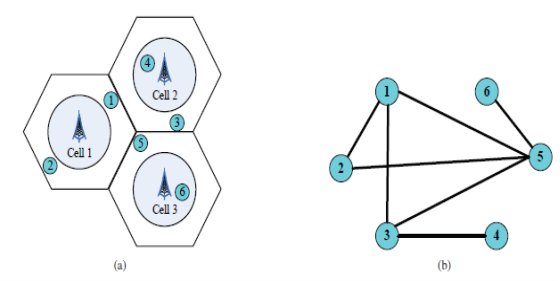Fig 5.1: An example of the graph-based framework. (a) 3-cell scenario (b) Interference graph construction.

### Phase-II Fine PRB Assignment

The first phase construction offers the network with a strategic planning for ICIC but the actual PRB allocation has not been done up till now. In the second phase, therefore we will come to a decision how to practically make the PRB assignment in the network given the interference graph. To reduce complexity, a heuristic algorithm is proposed here to perform a fine PRB allocation by taking account of the instantaneous channel quality.

As, major ICI is well looked after by the first stage in the second stage we consider simply SNR for ease by removing the interdependency matter of SINR

At this stage power is thought to be similarly disseminated over all PRBs as a result of the force allotment will be tended to in next segment. Thus, SNR for m on PRB n is computed by SNRnm=(Pmax/N)g(j-m)/N0, where j is the serving cell of user m. Let Rm be the arrangement of users why should permitted have the same PRB or in another word, the same shading with user m in the system. Then the PRB allocation can be described in Algorithm 1. Note that finding the necessary Rm is also included in this algorithm.

As indicated in Algorithm 1, the PRB assignment decision made to a user is resolved by the in a split second accomplished SNR as well as by a weighting element show by wm. The primary capacity of wm is to adjust the PRB distribution between cell-edge and cell-focus users of the system, i.e., higher weighting worth is given to cell-edge users as altogether reason they accomplish much lower SNRs contrasted with those of cell-focus users. Then again, the relative decency is likewise considered by making note of the quantity of PRBs as of now being used and in this way keeps the PRB designation from being selfishly abused by minority users in the system., Therefore, calculation 1 has the capacity perform PRB allotment that proficiently accomplishes a general execution change with great fairness amidst those two sorts of users in the system taking into account the weighted SNR . At that point the PRB designation can be demonstrated in Algorithm 1.

As shown in Algorithm 1, the PRB contribute to choice complete to a user is strong-minded not just with the right away achieved SNR other than too with a weighing factor indicated by wm. The first function of wm is to balance the PRB allocation among cell-edge and cell-centre users of the network, i.e., higher weighting value is given to cell-edge users as in general they achieve much lower SNRs compared to those of cell-centre users. Then again, the relative uniformity is additionally considered by making note of the quantity of PRBs officially involved and in this way keeps the PRB assignment from being selfishly abused by minority users in the system. Thus wm can be expressed by wm=w/|Nm|, where Nm is set of PRBs that are before allocated to user m,| ` | is the cardinality of a set (note that |Nm|= 1 if Nm=Ð¤), w = we when user m is a cell-edge user, otherwise w=wc and we>wc for return of the relatively low SNR received by cell-edge users.

Algorithm 1 is able to perform PRB allocation based on the weighted SNR that effectively achieves an overall performance improvement with good fairness among those two types of users within the network.

Therefore, radio resource distribution schemes effectively mitigated major ICI among cell-edge users of the network. On the other hand, cell-centre users are authorized to distribute PRBs by means of all users within the network (except their serving cells) and thereby may interfere with either cell-edge or cell-centre users of the adjacent cells depending on the PRB allocation by Algorithm 1, This interference determination be dealt by way of the following power allocation move towards.

## Power Allocation Approach

Subsequent to completing the PRB assignment, the estimations of all parallel variable aj mn in mathematical statement (4.4) are resolved. Hence, the first issue is diminished to streamlining issue where just transmission power pj mn is considered. Not at all like the radio asset assignment, the power portion is chosen separately in every cell and after that performed BSs in appropriated way. Accordingly, an appropriated force designation methodology is proposed in this segment with significance on execution advancement for cell-edge users.

### Total Power Distribution:

First and foremost we partition the aggregate transmission power of each cell into two sections: aggregate power of cell-edge users and cell-center users. Let Pj E and Pj C indicate the aggregate power distributed to cell-edge users and cell-center users in cell j, individually, and PjE+PjC=Pmax. Note that Pmax is assumed to be same for all BSs in the network. The determinations of PjE and PjC are based on following rules; relatively higher power is given in the direction of cell-edge users within order to balance the experienced large distance dependent path loss; and PjE(PjC) is proportional to the number of PRBs occupied with cell-edge (cell centre) users in cell j therefore, the relationship between PjE and PjC is expressed as shown in below equation (5.1)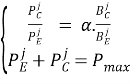………………….. (5.1)

Where BjC and BjE denote sets of total PRBs occupied by cell-centre and cell-edge users within cell j, respectively, and a(0

### Power Allocation for Cell-Centre Users

Keep in mind the issue detailing, the reason for comparison (4.4) is to restrictively expand the execution of cell-edge users and there is no improvement for cell-center users, while security of their execution is expressed as an essential breaking point. Subsequently, we essentially power the power designation to cell-center users by uniformly disseminating the aggregate power of cell-center users among their utilized PRBs as a part of every cell. Let pj(c).mn mean the power assigned to PRB n utilized by cell-center user m in cell j. At that point the neighborhood power assignment for cell-center users can choose by pj(c).mn demonstrated in comparison (5.2)

pj(c).mn = PjC/|BjC|,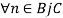………….. (5.2)

Note that user m in foreordained from the past PRB designation calculations by accomplishing aj.mn=1. Accordingly, in the accompanying we pass up a great opportunity m from the subscript variables of pj(c). Mn and streamline the expression as pj(c)n. To keep away from perplexity, we evacuate the general user file m from the subscript of all variables in the rest of this task.

### Power Allocation for cell-edge users

Given the stable PRB portion and force designation of cell-center users, the first improvement issue demonstrated in comparison (4.4) turns into a raised capacity of power of cell-edge users and can be deteriorated into J parallel sub-issues, where the ideal power allotment to cell-edge users is understood near to every BS of the system. Note that such sub-issues are characterized as a grouping of comparison (5.3), where just joint obstruction between cell-edge and cell-center users is taken into clarification. Let pj(e)n indicate the force assigned to PRB n utilized by a cell-edge user in cell j. Along these lines, the target of P2 for cell j is communicated as demonstrated in underneath mathematical statement (5.3)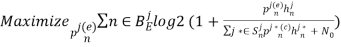……….. (5.3)

Where hjn (hj*n) indicates the joint channel impact on PRBn in cell j(j*) including the channel gain and path loss fading components presented in (3), i.e., hjn=g(j-m)nL_d(j-m)_ and Sjn is a set of neighbouring cells in the network (i.e., j*_=j,j*Ej), inside which PRB n is used with a cell-centre user at the moment. Above equation (5.3) is subject to the following constraints of the equation (5.4)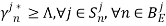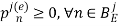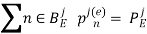……………….. (5.4)

Where yj*n is the SINR accomplished by a cell-center user of neighboring cell j* to whom p(e)n is the impedance source on PRB n, and É… is a suitable SINR edge decided to keep up elite of cell-center users in cell j*. The thought of P2 is to utilize power allotment to boost execution of cell-edge users under the imperative that execution of cell-center users is not generally give us. In other words, power allocation to cell-edge users to be conditionally optimized During order not to generate undesirable interference to cell-centre users in the network. In addition, here we take for granted that required information about the power allocation of cell-centre users in adjacent cells in known by each BS, which in fact are achievable in future wireless networks where specified links, such as the X2 interference in LTE, are built to connect BSs for necessary information exchange among them.

The Optimal Solution can be derived in below equation (5.5)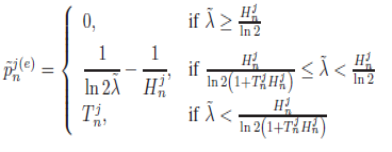………………………… (5.5)

As a barrier-constrained water-filling process the deviation can be also described graphically. By defining 1/Hjn as the base, 1/ln2~λ as the water level and 1/ln2~λ-Tjn as the barrier. The allocated power is indicated by the area (e.g., 1 and 2) below the water level and above both base and barrier. The allocated power is zero when either the base or barrier is above the water level. In addition, if the barrier (blue dashed line) is completely under the base, the base, the problem turns into a conservative water-fitting process.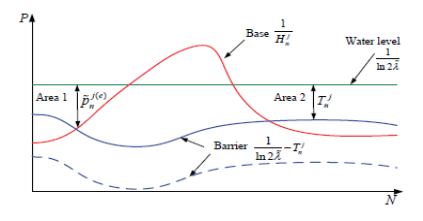Fig 5.2: An example of a barrier-constrained water-filling process.

Lastly, the optimal solution of ~λ and ~pj(e)n can be obtained by well known sub-gradient method, which can assurance a rapid convergence after a number of iterations. The difficulty of the future power distribution is O(||BjE||) which is self-governing of the number of cells in the network. Also this complexity is less than that of the general optimal power allocation where the complexity is O(N). For example, because the optimal power distribution is only executed intended for cell edge users and normally |BjE|

## Related Services

View all

### DMCA / Removal Request

If you are the original writer of this essay and no longer wish to have your work published on the UKDiss.com website then please:

Related Services